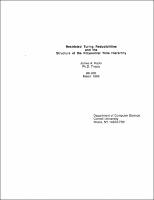## Restricted Turing Reducibilities and the Structure of the Polynomial Time Hierarchy##### Abstract
The polynomialtime many-one and Turing reducibilities, Karp and Cook reducibilities respectively, play an major role in computational complexity theory, particularly in the study of such classes as P, NP, the polynomial time hierarchy (PH), and PSPACE. In this thesis, we consider polynomial time Turing reducibilities with various restricted oracle access mechanisms such as restrictions on the number of queries allowed or requiring that all queries be made at once, in parallel. Such restrictions are related to polynomial time truth-table and bounded truth-table reducibilities. We focus mostly on classes of languages reducible to NP sets via these reducibilities. For any integer $k, P^{NP[k]}$ is the class of languages recognizable in polynomial time with $k$ queries to an oracle from NP. The query hierarchy, QH, is $\bigcup_{k} P^{NP[k]}$. The class $P^{NP[\log n]}$ is the set of languages recognizable with $O(\log n)$ queries. Two related hierarchies are the Boolean hierarchy Boolean hierarchy (BH) and the parallel query hierarchy $(\rm QH_{||})$. The BH, QH, and $\rm QH_{||}$ intertwine to form a rich structure within $P^{NP}$: NP $\cup$ co-NP $\subseteq P^{NP} \subseteq P^{NP} \subseteq \cdots \subseteq QH = QH_{||} = BH \subseteq P^{NP[\log n]} \subseteq P^{NP}.$ We show that the structure of these classes is closely tied to the existence of nonuniform algorithms for NP and co-NP languages and to the structure of the PH as a whole. We improve Mahaney's result for sparse Turing-complete sets for NP by showing that if there exists a sparse set $S \in NP$ such that co-NP $\subseteq NP^{S}$, then PH $\subseteq P^{NP[\log n]}$. We show that there are relativized worlds where this collapse is optimal, and thus we provide a clear distinction between the effects of a sparse many-one-complete set and a sparse Turing-complete set for NP. We prove that if the BH, QH, or $QH_{||}$ collapses, for instance, if $D^{P} =$co-$D^{P}$ or if $P^{NP[k]} = P^{NP[k+1]}$, then there exists a sparse set $S$ such that co-NP $\subseteq$NP^{S}$, and therefore the PH collapses to$P^{NP^{NP}[\log n]}$, a subclass of the$\Delta^{P}_{3}\$ level of the PH. Hence the structure of unsatisfiable Boolean formulas, co-NP, and the whole PH are all closely related to the structure of the BH and to the issue of how deterministic polynomial time algorithms can access the information from NP oracles.
1988-03
##### Publisher
Cornell University
##### Keywords
computer science; technical report
##### Previously Published As
http://techreports.library.cornell.edu:8081/Dienst/UI/1.0/Display/cul.cs/TR88-900
technical report